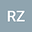Determination of the impulsive Dirac systems from a set of eigenvalues
•• Ran Zhang
Ran Zhang
Nanjing University of Science and Technology

Corresponding Author:[email protected]

Author Profile## Abstract

In this work, we consider the inverse spectral problem for the impulsive Dirac systems on $(0,\pi)$ with the jump condition at the point $\frac{\pi}{2}$. We conclude that the matrix potential $Q(x)$ on the whole interval can be uniquely determined by a set of eigenvalues for two cases: (i) the matrix potential $Q(x)$ is given on $\Big(0,\frac{(1+\alpha)\pi}{4}\Big)$; (ii) the matrix potential $Q(x)$ is given on $\Big(\frac{(1+\alpha)\pi}{4},\pi\Big)$, where $0<\alpha<1$.